• 频谱泄漏
千次阅读
2022-01-27 21:21:19

提示：文章写完后，目录可以自动生成，如何生成可参考右边的帮助文档

### FFT运算的加深理解——频谱泄露

还是接上一篇的例子，为了更好地描述，我们将其表示的物理意义添加上：

N FFT计算点数 8
Fs 采样率 8Hz
fi1 点频频率 1Hz
而且我们一般习惯于0频为中心位置,因此程序修改为这样

N = 8
fs = 8;
fi1 = 1;

N = 8
fs = 8;
fi1 = 1;
fcarr = 1*exp(j*2*pi*fi1/fs*[0:N-1]);

x_fft1 = fft(fcarr)/N;

stem([-4:3],abs(x_fft1))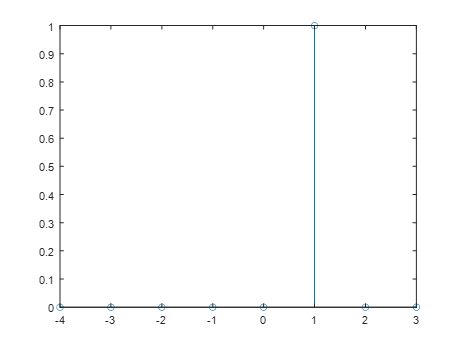这个图表示在1Hz处有一个幅度为1的点频

还有一个FFT分辨范围和分辨率的概念。
FFT的分辨范围是±fs/2内 。例如这里就是[-4,4)，

FFT的分辨率，是采样点总时间的倒数。例如这里，采样率是8Hz，点数是8个点，那么FFT的运算时间就是1s，1s的倒数就是1Hz。

我们将fi1 = 1.5;
一下子，所有的频率点都有幅值了，这就是频谱泄露。原本本该X=1.5Hz处有一条为1的幅值线变成了X = 2Hz处的幅值变成了0.64，且X=3Hz处的幅值也是0.64，这就是1.5Hz的幅值泄露到其他频率上去了，导致看起来其他频率都有信号一样，造成了分析的失真。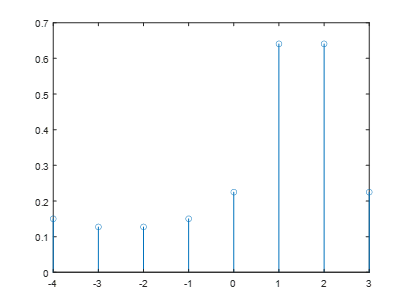（1）从信号连续性的角度来看，因为FFT运算是假设截取的信号是周期的，信号进行周期拓展之后会导致相位不连续，这样就会导致其他频率出现了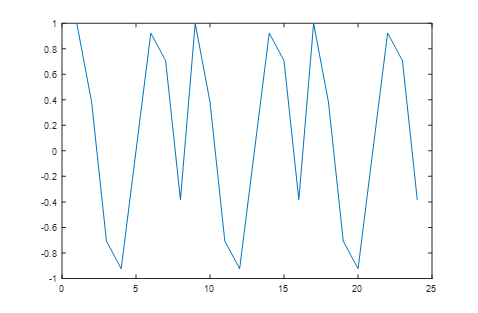（2）完全从 数学表达式来看

看W的定义：

W =  [exp(-j*2*pi/8*0),exp(-j*2*pi/8*1),exp(-j*2*pi/8*2),exp(-j*2*pi/8*3),exp(-j*2*pi/8*4),exp(-j*2*pi/8*5),exp(-j*2*pi/8*6),exp(-j*2*pi/8*7)]


可以看出
点频频率只能是1,2,3,4,5,6,7（或者1,2,3，-4，-3，-2,-1）才能完全与W正交，不是这些数计算肯定会有残留值。其他频率就会有残留值出现。

根据上面分析，除了点频是1,2,3，-4，-3，-2,-1Hz，其他频率必然会出现频谱泄露，导致幅值的失真。

失真最大的时候也就处于两个分辨频率的中间点，例如1Hz,2Hz中间点1.5Hz。
幅度只有正确的0.64。当点频为1.1Hz时，幅度为0.984。

整个频率区间[-4:3]Hz内，FFT幅度的变化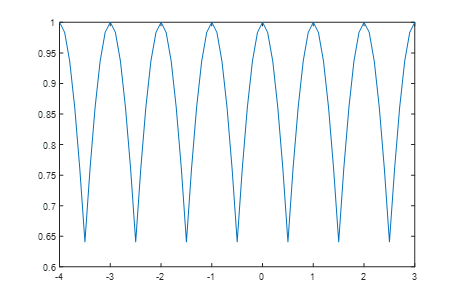用功率来表征的话，最多下降快4dB了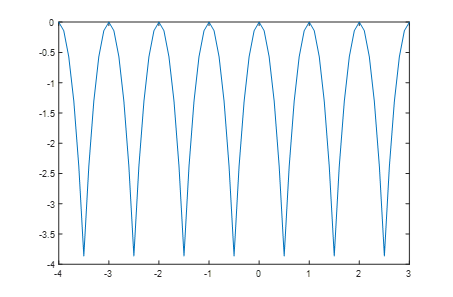频谱泄露会严重影响对频率的分析结果
1、 幅值变的比实际的低
2、 频率分析不准

但频谱泄露后，当前频率表征的幅度降低，但FFT表征的总功率并没有降低。
所有谱线的总功率和还是等于1

更多相关内容
• 简单的信号处理matlab实现，窗型和窗长对频谱泄漏影响的研究
• ## 频谱泄露

万次阅读 多人点赞 2016-05-10 14:13:52
fft在分析频谱分析的时候，会有下面四个方面的误差： （1）频谱混叠： 奈奎斯特定理已被众所周知了，所以几乎所有人的都知道为了不让频谱混叠，理论上采样频谱大于等于信号的最高频率。那和时域上联系起来的关系...

fft在分析频谱分析的时候，会有下面四个方面的误差：

（1）频谱混叠： 奈奎斯特定理已被众所周知了，所以几乎所有人的都知道为了不让频谱混叠，理论上采样频谱大于等于信号的最高频率。那和时域上联系起来的关系是什么呢？

采样周期的倒数是频谱分辨率，最高频率的倒数是采样周期。

设定采样点数为N，采样频率fs，最高频率fh，故频谱分辨率f=fs/N,而fs>=2fh，所以可以看出最高频率与频谱分辨率是相互矛盾的，提高频谱分辨率f的同时，在N确定的情况下必定会导致最高频率fh的减小；同样的，提高最高频率fh的同时必会引起f的增大，即分辨率变大。

（2）栅栏效应：由于dft是只取k=0,1,2,.......N-1,只能取到离散值，如果频谱之间相隔较大的话也许会将一些中间的信息丢失掉，而用fft计算dft是不可避免的，解决的办法就是增加采样点数N。这样频谱间隔变小，丢失信息的概率减小。

另外，增加0可以更细致观察频域上的信号，但不会增加频谱分辨率。

（3）频谱泄露：是由加窗函数引起的，同样是计算量的问题（用fft用dft必需要加窗函数），时域上的相乘，频域上卷积，引起信号的频谱失真，只有在很少的情况下，频谱泄露是不会发生的，大部分情况都会引起泄露。如x(n)=cos(2π/N),(n=0,1,2,3.....N-1,)  N点的fft则不会发生泄露，但2N，或N+1，N+2等均会引起失真，而引起失真可以从表达式上可以看出  X(K)=卷积以后的频谱在2π/N*k的      取样值，所以如果是2N的dft，为2π/2N*K,相当于N点dft结果各个值中间再取样了一个值，而2π/(N+2)*k,就与N点fft完全不一样了。解决办法，可以扩大窗函数的宽度（时域上的宽了，频域上就窄了，（时域频域有相对性），也就是泄露的能量就小了），或者不要加矩形的窗函数，可以加缓变的窗函数，也可以让泄露的能量变下。

因为泄露会照成频谱的扩大，所以也可能会造成频谱混叠的现象，而泄露引起的后果就是降低频谱分辨率。

频谱泄露会令主谱线旁边有很多旁瓣，这就会造成谱线间的干扰，更严重就是旁瓣的能量强到分不清是旁瓣还是信号本身的，这就是所谓的谱间干扰

所谓频谱泄露，就是信号频谱中各谱线之间相互影响，使测量结果偏离实际值，同时在谱线两侧其他频率点上出现一些幅值较小的假谱，导致频谱泄露的原因是采样频率和信号频率的不同步，造成周期采样信号的相位在始端和终端不连续。

设X(t)为实际信号，T0为信号周期，f0=1/T0为信号频率，Ts为采样周期，fs=1/Ts为采样频率，L是截取的周期数，N是采样点 数，L、N均为正整数，X(t)经过长度为LT0的时间窗后得到离散序列X(n)，必须满足采样频率和信号频率同步，即同步采样的要求： LT0/Ts=Nfs/f0。

当信号X(t)的频率f0是fs/N的整数倍时，这说明在处理长度NT内有信号的K个整周期。这时由X(t)构成的以NT为周期的周期性信号是连续 的。当信号X(t)的频率f0不是fs/N的整数倍时，则在NT的处理长度内，就不是恰好为信号周期的整数倍，有X(t)以NT为周期进行周期延拓所得到 的周期性信号就出现了不连续点，造成了频谱分量从其正常频谱扩展开来，就这样形成了频谱泄露现象。

在对信号做FFT分析时，如果采样频率固定不变，由于被采样信号自身频率的微小变化以及干扰因素的影响，就会使数据窗记录的不是整数个周期。从时域来 说，这种情况在信号的周期延拓时就会导致其边界点不连续，使信号附加了高频分量;从频域来说，由于FFT算法只是对有限长度的信号进行变换，有限长度信号 在时域相当于无限长信号和矩形窗的乘积，也就是将这个无限长信号截短，对应频域的傅里叶变换是实际信号傅里叶变换与矩形窗傅里叶变换的卷积。

当信号被截短后的频谱不同于它以前的频谱。例如，对于频率为fs的正弦序列，它的频谱应该只是在fs处有离散谱。但是，在对它的频谱做了截短后，结果 使信号的频谱不只是在fs处有离散谱，而是在以fs为中心的频带范围内都有谱线出现，它们可以理解为是从fs频率上泄露出去的，这种现象就是频谱泄露。泄 露现象对功率谱估计及正弦分量的检测均带来有害的影响，因为弱信号的主瓣很容易被强信号泄露到邻近的副瓣所淹没及畸变，从而造成谱的模糊与失真。通过 LABVIEW信号处理实验室可以看到当边界点不连续时出现的频谱泄露的情况如下图1所示：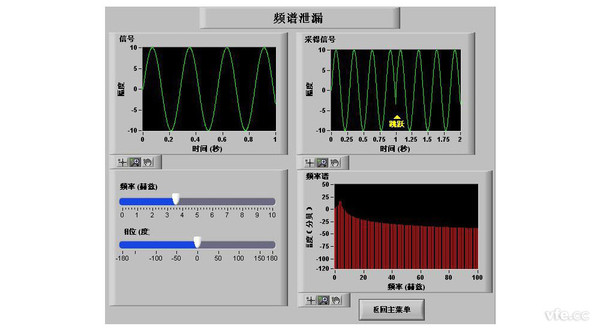图1 信号边界点不连续时

接下来举例说明以上的情况。假设连续信号X(t)的周期为T，现在对它进行采样，采样时间为t，采样N个点，那么T=N*t,因为f(t)的频率 f0=2*pi/T,同时又有T=N*t、fs=2*pi/t,则有f0=2*pi/N*t=fs/N。接着我们假设对一个周期采样N=32个点，则有 f0=fs/N;当对一个周期采样N1=64个点，那么N1=2*N，有f0=fs/N=fs/N1/2,即f0=2*fs/N1；同理当 N2=128，f0=4*fs/N2…

也就是说如果采样的不是整数倍的信号周期，那么这32个点、64个点、128个点....就不是在一个整周期内采到的，那么上面的等式也就不成立了， 因此也就发生了频谱泄露。如果原始信号的频谱成份与FFT中的谱线完全一致，这种情况下采样数据的长度为信号周期的整数倍，频谱中只有主瓣，没有出现旁瓣 的原因是旁瓣正处在窗函数主瓣两侧采样频率间隔处的零分量点。如果时间序列的长度不是周期的整数倍，窗函数的连续频谱将偏离主瓣的中心，频率偏移量对应着 信号频率和FFT频率分辨率的差异，这个偏移导致了频谱中出现旁瓣，所以窗函数的旁瓣特性直接影响着各频谱分量向相邻频谱的泄露宽度。下图2是信号边界连 续时的频谱图，可以看到此时频谱未发生泄露。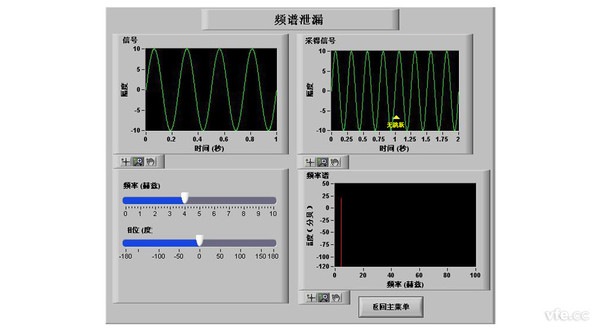图2 信号边界点连续时

因此，综上所述，当采样同步时，窗口宽度等于整数个信号周期，矩形框的过零点与离散频点正好对齐，没有泄露。采样不同步时，窗口宽度不是整数个信号周期，谐波频谱分布不再是一条谱线而是在整个频域内分布，频谱之间相互干扰，出现频谱泄露。

由以上分析可以看出，采样不同步是造成频谱泄露的根本原因，减少采样的同步误差是抑制频谱泄露的根本措施。

三、消除频谱泄露的处理方法

01选择合适的窗函数

为减少频谱能量泄露，可采用不同的截取函数对信号进行截断，截断函数称为窗函数。 泄露与窗函数频谱的两侧旁瓣有关，如果两侧旁瓣的高度趋于零，而使能量相对集中在主瓣，就可以较为接近于真实的频谱，为此，在时间域中可采用不同的窗函数 来截断信号，应用在谐波测量中的窗函数很多，不同的窗函数对谐波测量的影响各不相同，即使同一个窗函数，参数选择不一样，影响也不一样，以下是6种常用的 窗函数及特性：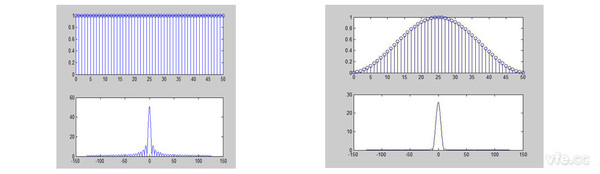矩形窗汉宁窗(Hanning)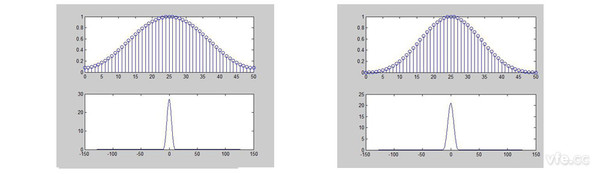汉明窗(Hamming) 布莱克曼窗(Blackman)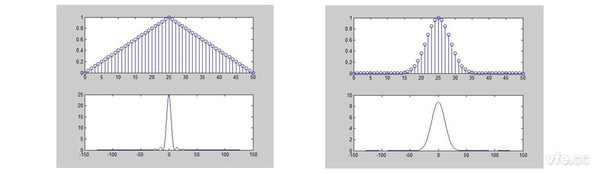Bartlett窗(三角窗) 凯泽窗(Kaiser)

选择合适的窗函数对于减少频谱能量泄露非常有效，以下是窗函数的选择建议：

如果在测试中可以保证不会有泄露的发生，则不需要用任何的窗函数；

如果测试信号有多个频率分量，频谱表现的十分复杂，且测试的目的更多关注频率点而非能量的大小。在这种情况下，需要选择一个主瓣够窄的窗函数，汉宁窗是一个很好的选择；

如果测试的目的更多的关注某周期信号频率点的能量值，那么其幅度的准确性则更加的重要，可以选择一个主瓣稍宽的窗；

如果检测两个频率相近幅度不同的信号，用布莱克曼窗；

如想要灵活的通过窗函数的形状来控制频谱波纹或阻带衰减指标，则选择凯泽窗；

如果被测信号是随机或者未知的，选择汉宁窗。

02加长傅里叶时间窗长度

傅里叶时间窗长度就是参与傅里叶变换的数据点数，参与变换的数据点数越多，频谱泄露越小。对于快速傅里叶变换FFT，要求数据点数必须为2的N次幂，而对于普通离散傅里叶变换DFT则无此限制。

以下是不同采样次数和窗宽对应的频谱泄露，从对比图上可以看出，当采样次数N和窗宽L同时增大时，频谱泄漏减小。不同采样次数和窗宽对应的频谱泄露

03利用频率同步装置减少频谱泄露

利用硬件装置实现频率同步的装置很多，其中下图3所示的是利用数字式锁相器(DPLL)实现频率同步的框图。图中带通滤波器用来滤除噪声干扰，数字式 相位比较器把取自系统电压信号的相位和频率与锁相环输出的同步反馈信号进行相位比较。当失步时，数字式相位比较器输出与两者相位差和频率差有关的电压，经 滤波后控制并改变压控振荡器的频率，直到输入的频率和反馈信号的频率同步为止。一旦锁定，便将跟踪输入信号频率变化，保持两者的频率同步，输出的同步信号 去控制对信号的采样和加窗函数。图3 频率同步数字锁相装置框图

此种采用锁相环技术实现硬件同步采样的方法尽管可以在一定程度上减小频谱泄露，但其加大了硬件复杂度。

04利用采样频率自适应软件算法来减少频谱泄露

对于实际的电力信号，其频率的变化一般是比较缓慢的，相邻的几个周波的频率变化很小，在对其进行频谱分析时，针对电力信号这个特点，采用软件采样频率 自适应算法。首先以基波频率50HZ为采样基点，然后通过软件算法得到信号的实际频率，用实际频率自动的调整采样时间，可以减小同步误差，提高精度。

对于实际电力信号，软件采样频率自适应算法如下：

(1)设定采样间隔为Ts=78.125us(采样频率fs=12.8kHz)，采样2×512+256点；

(2)根据采样序列和采样间隔，利用改进的过零修正法计算信号的实际频率f0；

(3)由实际频率f0调整新的采样时间间隔，可使新的采样时间窗是T0=1/f0的整数倍，并采样2×512+256点；

(4)利用过采样法进行FFT运算得到64点(N=512而只计算64点)结果，输出基波以及各次谐波数据；

(5)将2×512+256点采样序列和新的采样间隔作为参数，返回第(2)步。

本算法主要有以下特点：

(1)在第2步中采用数字滤波过零修正法。仅仅采用一般的过零线性化来计算采样频率所得到f0的误差还是较大的，由于干扰或者某些因素影响，有时甚至 会出现错误。为了解决这个问题，对采样序列进行数字滤波，一般来说基频信号最强，受其他谐波泄露的影响也最小，通过数字滤波器除基频以上的频率成分，保证 基波附近频率通过，并采用16位A/D转换芯片，可明显降低频率计算误差。

(2)在第3步中通过软件实现对采样时间间隔(采样频率)的调整，在实际应用中，采用DSP芯片对采样时间的确定可以精确到0.01us，有很好的精度保证。

(3)第4步中利用过采样法进行FFT运算，可以减小混叠误差，或降低对混叠滤波器的 要求。如果进行FFT运算的序列不是充分的，其fs/2以上的频率成分就会折叠到0~fc段内。对于电力信号，要求分析的最高为64次的谐 波，fc=3.2kHz，则fs~fc，2fs~3fc，3fs~5fc，4fs~7fc段频谱会折叠到0~fc段内引起折叠误差。并且在fs/2附近折 叠误差最大；但当fc一定时，提高fs会减小误差，因此，在进行信号分析时，可用过采样方法减小混叠误差。

展开全文• 在正弦相位调制(SPM)干涉仪中,若调制频率或者采样频率发生变化将使干涉信号出现频谱泄漏,减小了谐波分量的幅值,在测量结果中引入了误差。对频谱泄漏的产生及其对测量精度的影响进行了理论分析,获得了频谱泄漏引入...
• 一、频谱泄露 1. 定义 频谱泄露会导致主谱线旁边有很多旁瓣，这会造成谱线间的干扰，严重的可能是旁瓣能量强到分不清哪个是主瓣，这就是所谓的谱间干扰。 （参考：FFT造成的频谱混叠，栅栏效应，频谱泄露，谱间...

# 一、频谱泄露

### 1. 定义

频谱泄露会导致主谱线旁边有很多旁瓣，这会造成谱线间的干扰，严重的可能是旁瓣能量强到分不清哪个是主瓣，这就是所谓的谱间干扰。

### 2. 产生原因

信号被非周期截断，导致延拓信号和原信号的相位不连续，那么做 FFT 变换，得到的频谱就会发生频谱泄露。所以发生频谱泄露的原因是：信号被非周期截断/加窗。

如下图：图 1 的第 1 个子图是信号被周期截断，第 2 个子图是被非周期截断，它们的傅里叶变换见图 2。可以看到第 1 个子图的 FFT 能量集中在某个频率，而第 2 个子图在主频点附近的频点上，也有许多不可忽视的能量，即主频点能量泄漏到附近频点上。

（参考：如何理解频谱泄露？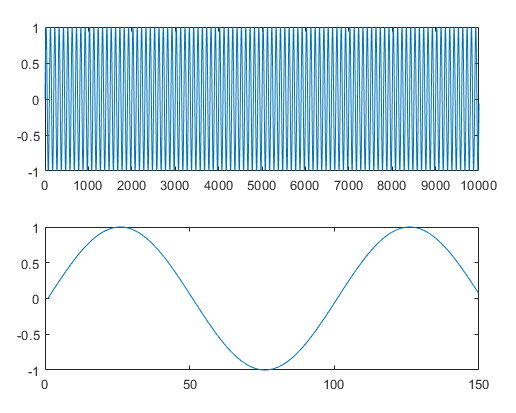图1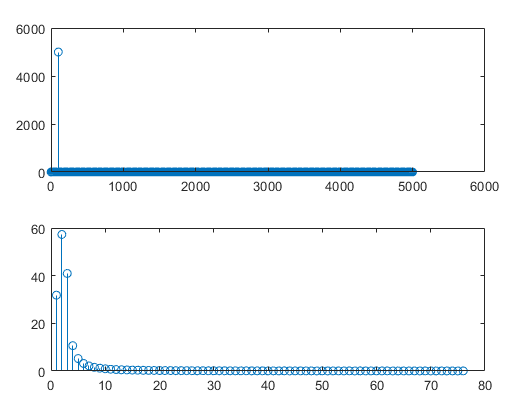图2

（参考：频谱泄露和加窗

### 3. 解决办法

时域中的突变点会对 FFT 之后的频谱有明显的影响，并且频谱泄露的程度取决于时域信号边界的不连续程度，解决办法是：周期截断信号。

但很难做到周期截断，只能引入分窗算法，通过加窗的方式使截断加窗后的信号尽可能地接近周期信号（只不过一个窗信号就是一个周期）

注：加窗只能减少频谱泄露，但不能解决频谱泄露！

# 二、举个栗子

%% 频谱泄露
clear; clc; close all; warning off;

%% 参数设置
fs = 100;  % 采样频率（满足奈奎斯特采样定理：要大于信号最大频率的两倍！！！）
L = 5;  % 时间长度
t = 0 : 1/fs : L;  % 时间坐标
t(1) = [];

%% 生成信号
f = 6;  % 信号频率
s0 = sin(2 * pi * f * t);

% 加窗
winFunc = hamming(length(s0))';  % 汉明窗
s1 = s0 .* winFunc;

%% FFT
numfft = 1024;  % FFT点数
f = (0 : numfft/2-1) / numfft * fs;  % 频率（只取正频部分）

s_fft = fft(s0, numfft);
p = abs(s_fft(1:numfft/2)) / numfft;  % 功率（只取正频部分）

s1_fft = fft(s1, numfft);
p1 = abs(s1_fft(1:numfft/2)) / numfft;  % 功率（只取正频部分）

%% 作图
figure(1);
subplot(2, 1, 1);
plot(s0, 'linewidth', 1.5); title('原始信号时域波形'); axis('tight'); ylim([-1.2, 1.2]);
subplot(2, 1, 2);
plot(s1, 'linewidth', 1.5); title('加窗信号时域波形'); axis('tight'); ylim([-1.2, 1.2]);

figure(2);
sp1 = subplot(2, 1, 1);
plot(f, p, 'linewidth', 1.5); axis('tight'); title(['原始信号s0的', num2str(numfft), '点傅里叶变换']);
sp2 = subplot(2, 1, 2);
plot(f, p1, 'linewidth', 1.5); axis('tight'); title(['加窗信号s1的', num2str(numfft), '点傅里叶变换']);
linkaxes([sp1, sp2], 'x');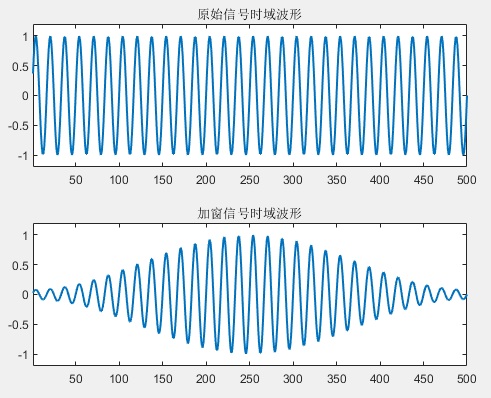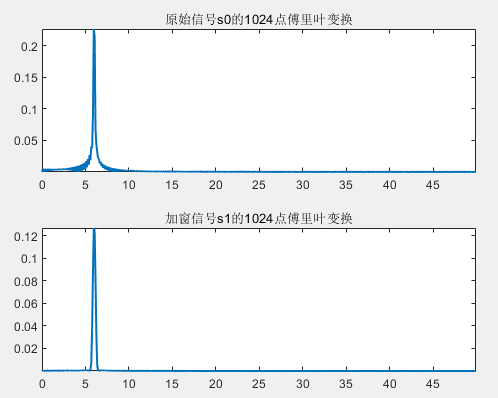局部放大之后：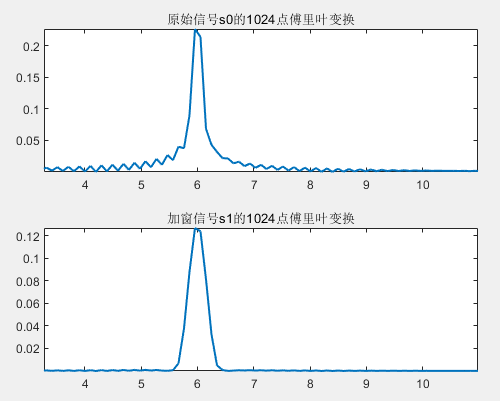从上图分析得到，原始信号是被非周期截断的，所以得到的 FFT 发生了频谱泄露现象，体现在主频附近一些频点有较高的能量。而经过加窗处理后的信号类似于周期信号，所以加窗信号的 FFT 的频谱泄露现象减少（但不是消除！）。

原理见下图：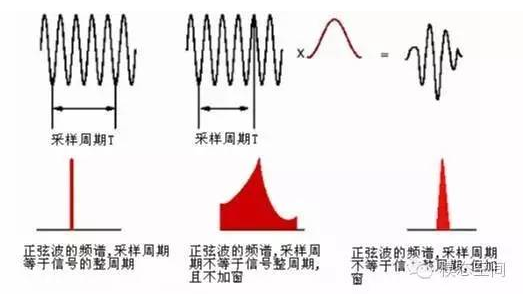（参考：频谱泄露和吉布斯现象

展开全文matlab 开发语言
• 信号的频域分析一、时域加窗频谱泄露产生频谱泄露的原因是什么？如何抑制这一现象？二、频率分辨率频率分辨率如何计算？怎样提高频率分辨率？三、频域采样栅栏效应如何缓解栅栏效应？四、MATLAB代码 一、时域加窗 ...

# 一、时域加窗

现实生活中的信号大部分是连续的，通过对连续的信号进行采样得到散时间信号，但是计算机所能处理的数据都是有限长的，因而我们可以对原始序列做加窗处理使其成为有限长序列。
以矩形窗为例，其时域表达式为：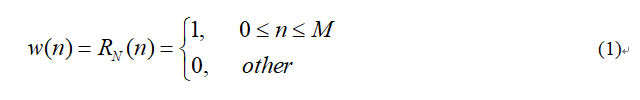式(1)中， N = M + 1 N=M+1 ，为矩形窗的长度。

对无限长序列进行加窗处理，就是对序列在时域上乘以一个窗函数。
由卷积定理可以得到，时域的相乘等于频域的卷积。

设仿真信号的时域表达式为：
x ( t ) = A 0 ∗ c o s ( 2 π f 0 t ) + A 1 ∗ c o s ( 2 π f 1 t ) x(t)=A_{0}*cos(2πf_{0}t)+A_{1}*cos(2πf_{1}t)
x ( t ) x(t) 做傅里叶变换（FT）的频域表达式为：
X ( j Ω ) = A 0 π δ ( Ω + Ω 0 ) + A 0 π δ ( Ω − Ω 0 ) + A 1 π δ ( Ω + Ω 0 ) + A 1 π δ ( Ω − Ω 0 ) X(jΩ)=A_{0}πδ(Ω+Ω_{0})+A_{0}πδ(Ω-Ω_{0})+A_{1}πδ(Ω+Ω_{0})+A_{1}πδ(Ω-Ω_{0})
连续信号 x ( t ) x(t) 的波形及频谱如图1所示。连续信号 x ( t ) x(t) 经过采样后，得到的离散时间的表达式为：
x [ n ] = x ( t ) ∣ t = n T s x[n]=x(t)|_{t=nT_{s}}
离散序列 x [ n ] x[n] 做离散时间傅里叶变换（DTFT）的频域表达式为：
X ( e j w ) = 1 T s ∑ k = − ∞ ∞ X ( j w T s − j k 2 π T s ) X(e^{jw})=\frac{1}{T_{s}}\sum_{k=-∞}^{∞}X(j\frac{w}{T_{s}}-jk\frac{2π}{T_{s}})
离散序列 x [ n ] x[n] 的波形及频谱如图2所示。矩形窗函数 w [ n ] w[n] 做离散时间傅里叶变换（DTFT）的频域表达式为：
W ( e j w ) = e − j w ( N − 1 ) / 2 ∗ s i n ( w N / 2 ) s i n ( w / 2 ) W(e^{jw})=e^{-jw(N-1)/2} *\frac{sin(wN/2)}{sin(w/2)}

矩形窗函数 w [ n ] w[n] 的波形及频谱如图3所示。离散序列 x [ n ] x[n] 与窗函数 w [ n ] w[n] 的卷积为：
V ( e j w ) = 1 2 π ∫ − π π X ( e j θ ) W ( e j ( w − θ ) ) d θ = A 0 2 W ( e j ( w + w 0 ) ) + A 0 2 W ( e j ( w − w 0 ) ) + A 1 2 W ( e j ( w + w 0 ) ) + A 1 2 W ( e j ( w − w 0 ) ) V(e^{jw})=\frac{1}{2π}\int_{-π}^{π}X(e^{jθ})W(e^{j(w-θ)})dθ=\frac{A_{0}}{2}W(e^{j(w+w_{0})})+\frac{A_{0}}{2}W(e^{j(w-w_{0})})+\frac{A_{1}}{2}W(e^{j(w+w_{0})})+\frac{A_{1}}{2}W(e^{j(w-w_{0})})
截断后的离散序列 v [ n ] v[n] 的波形及频谱如图4所示。## 频谱泄露信号的频率成分包括1MHz和1.05MHz，1MHz对应的幅值为1，但是1.05MHz的幅值减小了，且在其他频率点上都有不小的幅值。这就是出现了频谱泄露的现象。

### 产生频谱泄露的原因是什么？

由于计算机只能处理有限长的数据，所以需要对采集的信号进行截断，相当于对原始信号做了加窗处理。对信号加窗就是对信号在时域上乘以一个窗函数，时域的乘积对应频域的卷积，而窗函数的频域包括主瓣和旁瓣，旁瓣造成了信号频谱的泄漏。频域泄漏不可避免，只能减小。

### 如何抑制这一现象？

可以取更长的数据点，与原始数据越接近越好，但缺点就是运算量加大；
可以选择窗谱的旁瓣能量较小的窗函数。
典型的窗函数中，矩形窗的频率分辨率最高，旁瓣泄露最大。

# 二、频率分辨率

## 频率分辨率如何计算？

为了便于理解什么是频率分辨率，可以将频率分辨率划分为两种类型，一种是波形频率分辨率（Waveform Frequency Resolution，简记为波形分辨率），也称为视觉频率分辨率，另一种则为FFT频率分辨率（简记为FFT分辨率）。

波形分辨率：在频谱图中，两个频率可以被分辨率的最小间隔，与原始信号的时间长度有关。
△ R w = 1 T △R_{w}=\frac{1}{T}
其中， T T 为原始数据的时长。

FFT分辨率：在频谱图中的数据点数，跟信号做FFT计算时的点数有关。
△ R f f t = F s N f f t △R_{fft}=\frac{F_{s}}{N_{fft}}
其中， F s F_{s} 为采样频率， N f f t N_{fft} 为信号做FFT计算时的点数。

## 怎样提高频率分辨率？

例如，有一个复合信号的时域表达式为 x ( t ) = c o s ( 2 π f 1 t ) + c o s ( 2 π f 2 t ) x(t)=cos(2πf_{1}t)+cos(2πf_{2}t)
其中 f 1 = 1 M H z f_{1}=1MHz f 2 = 1.05 M H z f_{2}=1.05MHz x ( t ) x(t) 的波形及频谱如图6所示。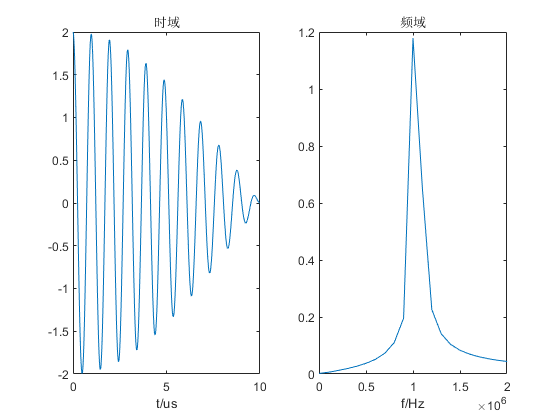由上图中的频谱可以发现，在1MHz附近两个频率出现混叠，无法有效区分1MHz和1.05MHz，说明频率分辨率不够。

对时域数据进行补零，能否改变频率分辨率呢？例如在原始数据点后面再补充6000个数值为0的点，对信号本身数据没有影响，只是增加了参与FFT计算的数据点数，得到信号的波形及频谱如图7所示。通过对原始数据进行补零操作，在频谱图中的数据点更密集了。但是仍然无法区分1MHz和1.05MHz，由此证明信号的波形频率分辨率与参与FFT计算的数据点数 N f f t N_{fft} 无关，只与原始数据的时长 T T 有关。在时域上补零等价于频域上进行插值，由此增加了频率的点数，使得频谱曲线变得更光滑，即增加了FFT频率分辨率。

为了有效区分1MHz和1.05MHz，必须延长原始数据的时长以提高波形分辨率。以相同的采样频率对原始信号进行采样，采集7000个数据点。得到信号的波形及频谱如图8所示。有图8可见，1MHz和1.05MHz可以被区分开，但是也出现了频谱泄露现象。此时信号的波形分辨率为： △ R w = 1 70 u s ≈ 14 K H z △R_{w}=\frac{1}{70us}≈14KHz ，小于1MHz和1.05MHz之间的距离 50 K H z 50KHz

为了减小频谱泄露，对原始信号取更长的数据点，采集8000个数据点。得到信号的波形及频谱如图9所示。由图9可知，1MHz和1.05MHz对应的幅值均为1，且可以有效的区分开，此时信号的FFT分辨率为： △ R f f t = F s 8000 = 12.5 K H z △R_{fft}=\frac{F_{s}}{8000}=12.5KHz ，刚好是1MHz和1.05MHz的公约数，即 1 M H z = 12.5 K H z × 80 1MHz=12.5KHz×80 1.05 M H z = 12.5 K H z × 84 1.05MHz=12.5KHz×84
所以取合适长度的数据点可以减轻频谱泄露。

# 三、频域采样

周期序列的DFS的系数 X ( k ) X(k) x ( n ) x(n) 的一个周期的 Z Z 变换在单位圆的 N N 个均匀点上的抽样值相等，这就是频域采样。

频域采样定理：
N ≥ L N≥L ，即DFT计算的频域采样点数大于等于信号的长度时，频域采样不会造成时域混叠。
有限长序列 x ( n ) x(n) Z Z 变换为：
X ( Z ) = ∑ n = 0 N − 1 x ( n ) Z − n = ∑ n = 0 N − 1 [ 1 N ∑ k = 0 N − 1 X ( k ) W N − k n ] Z − n = 1 − Z − N N ∑ k = 0 N − 1 X ( k ) 1 − W N − k Z − 1 X(Z)=\sum_{n=0}^{N-1}x(n)Z^{-n}=\sum_{n=0}^{N-1}[\frac{1}{N}\sum_{k=0}^{N-1}X(k)W_{N}^{-kn}]Z^{-n}=\frac{1-Z^{-N}}{N}\sum_{k=0}^{N-1}\frac{X(k)}{1-W_{N}^{-k}Z^{-1}}
其中， W N − k n = e j 2 π k n N W_{N}^{-kn}=e^{j\frac{2πkn}{N}} W N − k = e j 2 π k N W_{N}^{-k}=e^{j\frac{2πk}{N}}

## 栅栏效应

在进行DFT计算时需要对信号的频域进行采样，由于采样间隔为 △ w = 2 π N △w=\frac{2π}{N} ，得到的频谱图都是由一根根离散的谱线组成，就像透过栅栏观看外景。## 如何缓解栅栏效应？

增加频域采样点数N（不改变时域数据的情况下，在时域数据末端添加一些零值点，使得谱线更密），可缩小谱线间距，减轻栅栏效应。

# 四、MATLAB代码

%% 1000个数据点的波形及频谱
clc;
clear;
close all;

Fs = 100e6;             % 采样频率
f1 = 1e6;f2 = 1.05e6;   % 信号的频率
T = 1/Fs;               % 采样周期
L0 = 1000;              % 信号长度
L = 1000;               % 数据长度
t0 = (0:L0-1)*T;        % 信号时间序列
t = (0:L-1)*T;          % 数据时间序列
x = cos(2*pi*f1*t0)+cos(2*pi*f2*t0);  % 原始信号

% FFT
[f1,A1] = PinPu(x,Fs);
figure(1)
subplot(1,2,1);plot(t*1e6,x);
xlabel('t/us');title('时域');
subplot(1,2,2);plot(f1,A1);
xlabel('f/Hz');title('频域');xlim([0 2e6]);

%% 出现了频谱泄露现象
clc;
clear;
close all;

Fs = 100e6;             % 采样频率
f1 = 1e6;f2 = 1.05e6;   % 信号的频率
T = 1/Fs;               % 采样周期
L0 = 7000;              % 信号长度
L = 7000;               % 数据长度
t0 = (0:L0-1)*T;        % 信号时间序列
t = (0:L-1)*T;          % 数据时间序列
x = cos(2*pi*f1*t0)+cos(2*pi*f2*t0);  % 原始信号
% FFT
[f1,A1] = PinPu(x,Fs);
figure(1)
subplot(1,2,1);plot(t*1e6,x);
xlabel('t/us');title('时域');
subplot(1,2,2);plot(f1,A1);
xlabel('f/Hz');title('频域');xlim([0 2e6]);

%% 8000个数据点的波形及频谱，提升了频率分辨率。
clc;
clear;
close all;

Fs = 100e6;             % 采样频率
f1 = 1e6;f2 = 1.05e6;   % 信号的频率
T = 1/Fs;               % 采样周期
L0 = 8000;              % 信号长度
L = 8000;               % 数据长度
t0 = (0:L0-1)*T;        % 信号时间序列
t = (0:L-1)*T;          % 数据时间序列
x = cos(2*pi*f1*t0)+cos(2*pi*f2*t0);  % 原始信号
% FFT
[f1,A1] = PinPu(x,Fs);
figure(1)
subplot(1,2,1);plot(t*1e6,x);
xlabel('t/us');title('时域');
subplot(1,2,2);plot(f1,A1);
xlabel('f/Hz');title('频域');xlim([0 2e6]);



# 参考文献

展开全文数字信号处理 频率分辨率
• 如上图所示，在采样时间内，被采样信号的频率是基波频率(fsf_sfs​)的三倍，从下面DFT的幅值可以看出，因为周期是采样时间整数倍的缘故，所以没有发生频谱泄露现象。 补充： fanalysis = fs∗mN &...算法
• ## 频谱泄露与窗函数

千次阅读 2020-10-18 16:27:12
频谱泄露与窗函数  今天，我想谈的是数字信号处理中的两个重要的问题，什么是频谱泄露，以及什么是窗函数，为什么使用窗函数。 文章目录频谱泄露与窗函数什么是频谱泄露一、频谱泄露是什么？二、窗函数的目的 ...matlab 数字信号处理
• 若实际应用中，待处理信号不满足周期性，则会引发“频谱泄露”问题。 频谱泄露的具体原因以及公式推导这里不再赘述，已经有很多大佬对此展开过讨论。简单来说，是由于周期延拓后信号的不连续性导致的。这里做个简单...算法 matlab 数字信号处理
• 信号为无限长序列，运算需要截取其中一部分（截断），于是需要加窗函数，加了窗函数相当于时域相乘，于是相当于频域卷积，于是频谱中除了本来该有的主瓣之外，还会出现本不该有的旁瓣，这就是频谱泄露！为了减弱...
• 理想情况 假设一个信号由两个频率不一样的余弦波组成cos(2πft)=cos(2πf1t)+cos(2πf2t)cos(2\pi ft)=cos(2\pi f_1t)+cos(2\pi f_2t)cos(2πft)=cos(2πf1​t)+cos(2πf2​t)组成，其中f1=50Hz,f2=65Hzf_1=50Hz,f_2...
• ## 关于频谱泄露

千次阅读 2018-04-02 12:26:54
结果发现是无限连续信号在进行DFT时，由于截取效应所产生的频谱泄露。解决方案：1）加个窗函数。于是，我加上了hamming窗，旁瓣是消息了，但是加窗相当于加了个滤波器，对最后的指标估计有影响。2）用连续傅里叶变换...
• 我们分析的信号，如果只含整数次谐波的话，用FFT分析信号的频谱和相位是非常准确的，如果信号含有确定的间谐波，比如信号含有60HZ和65HZ的频率，那我们也可以准确的分析出信号的频谱和相位，我们只要用矩形窗截取10...
• ## 补零与频谱泄露

千次阅读 2017-11-28 15:02:13
频谱泄露频谱泄露与傅里叶变换尤其是离散时间傅里叶变换有关，对于频谱泄露，通常的解释是这样的： 　信号为无限长序列，运算需要截取其中一部分（截断），于是需要加窗函数，加了窗函数相当于时域相乘，于是相当于...
• %加海明窗，幅值相等恢复系数为1.852 测试参数： 采样率为1000（非周期采样），采样1024点计算FFT，信号周期50Hz，测试环境matlab R2018a 无频谱泄漏波形： 未加窗未滤波的波形： 未加窗加滤波的波形： 结论： 从...算法
• 频谱泄露1.1 什么是频谱泄露1.2 频谱泄露的影响1.3 如何减少频谱泄露2. 栅栏效应2.1 什么是栅栏效应2.2 怎样减小栅栏效应 1. 频谱泄露 1.1 什么是频谱泄露 实际情况下，时频谱都被限制为有限长序列。在处理过程中...数字信号处理 语音识别
• DFT泄漏(频谱泄漏) 作者：冰三点水 本贴简单介绍DFT泄漏的原理以及处理方法，转帖请尊重原创 http://blog.csdn.net/u013608300/article/details/78704762。 DFT(Discrete Fourier Transform，离散傅立叶变换)中...
• DFT中时域补零，频域对于单周期的FFT会更加细致，因为本来的DTFT就是对补零的部分取零的，补零反而能使DFT的采样更精细，何乐而不为呢？当然，补零对周期信号的DFT是一定存在影响的，毕竟周期变了。...
• 什么是泄漏？ 在做信号处理时，经常涉及到“泄漏”。那泄漏是什么，是什么原因造成了泄漏呢？在这将告诉您答案。   1.信号截断 一次FFT分析截取1帧长度的时域信号，这1帧的长度总是有限的，因为FFT分析一次只能...
• 近年来针对频谱泄漏抑制方法的研究受到...根据频谱泄漏类型,分别从短程频谱泄漏和长程频谱泄漏两方面,综合论述了现有频谱泄漏抑制方法的基本思想、算法原理和特点,最后对频谱泄漏抑制方法的发展前景进行了探讨和展望。
• (二) 频谱泄漏与加窗 频谱泄漏也做FFT时最常见的一个问题，举一个更简单的例子： 设信号 w(t)=sin(2πft)，f=10Hzw(t)=sin(2πft)，f=10Hzw(t)=sin(2πft)，f=10Hz，对 w(t)w(t)w(t)进行离散采样，采样率为200Sa/s...快速傅立叶变换
• ## 什么是频谱泄露

千次阅读 2016-04-10 18:02:05
但是，在利用DFT求它的频谱时，对时域做了截断，结果使信号的频谱不只是在fs处有离散谱，而是在以fs为中心的频带范围内都有谱线出现，它们可以理解为是从fs频率上“泄漏”出去的，这种现象称 为频谱泄漏”。  ...
• ## FFT频谱泄露和加窗 （二）

万次阅读 多人点赞 2017-05-20 23:36:54
图11： 测量非整数个周期（上图）将频谱泄漏添加至FFT（下图）。 可通过加窗来尽可能减少在非整数个周期上进行FFT产生的误差。 数字化仪采集到的有限序列的边界会呈现不连续性。加窗可减少这些不连续部分的幅值。...
• ## 深入浅出的理解频谱泄露

万次阅读 多人点赞 2015-01-28 22:32:04
这个世界上有很多概念，本来非常简单，可是，被一些学者一解释，就变得复杂了。...　频谱泄露与傅里叶变换尤其是离散时间傅里叶变换有关，对于频谱泄露，通常的解释是这样的： 　信号为无限长序列，
• ## 频谱泄露和吉布斯现象

千次阅读 多人点赞 2018-12-27 09:10:07
对比一下正确的频谱与发生泄漏频谱，如下图所示，可以看出，泄漏后的频谱的幅值更小，频谱拖尾更严重。当截断后的信号不为周期信号时，就会发生泄漏。而现实世界中，在做FFT分析时，很难保证截断的信号为周期信号...
• 讨论了频谱泄漏对傅里叶变换轮廓术测量精度的影响。 由于用傅里叶变换轮廓术进行三维面形测量时, 测得的变形光场是空间有限函数, 故离散傅里叶变换时先要进行周期拓展, 如果拓展周期选择不当, 拓展后的条纹将不连续,...
• 1. 频谱混叠 产生原因：采样频率不满足奈奎斯特采样定理，即采样频率过低，不满足采样频率高于信号最高频率的两倍的条件。 解决方法： （1）增加时域采样点数，即提高采样频率； （2）或者在采样之前加一个抗...fpga开发 网络协议
• 什么是频谱泄露？ 一些人可能会说，频谱泄露就是频谱中除了本来该有的主瓣之外，还会出现本不该有的旁瓣；一下子主瓣、旁瓣这些概念都来了，这些一些很抽象的概念，搞得我们很难去理解。其实频谱泄露就一句话：原来...matlab 傅立叶分析...